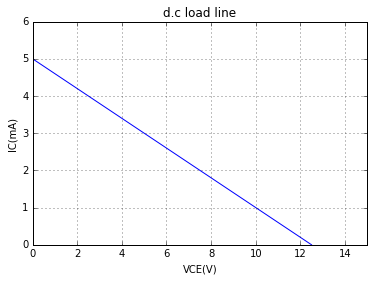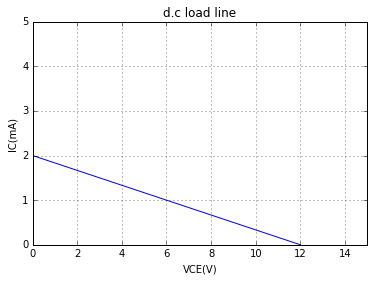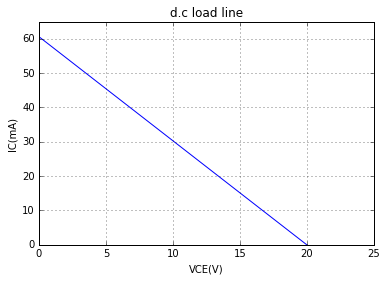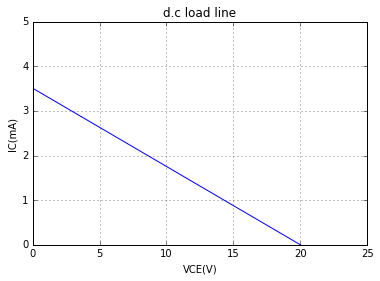## Example 8.1: Page number 147-148¶

In :
#Variable declaration
Signal=500.0;                    #Signal voltage in V
Rin=20.0;                        #Input resistance in Ω
Rout=100.0;                      #Output resistance in Ω
alpha_ac=1.0;                    #current amplification factor

#Calculation
I_E=(Signal/1000)/Rin;        	#Input current in mA
I_C=I_E*alpha_ac;               #Output current in mA
Vout=I_C*R_C;                   #Output voltage in V
Av=Vout/(Signal/1000);          #Voltage amplification

#Result
print("The voltage amplification = %d. "%Av);

The voltage amplification = 50.


## Example 8.2: Page number 150¶

In :
#Variable declaration
I_E=1;                            #Emitter curent in mA
I_C=0.95;                         #Collector current in mA

#Calculation
I_B=I_E-I_C;                      #Base current in mA

#Result
print("The base current = %.2f mA "%I_B);

The base current = 0.05 mA


# Example 8.3: Page number 150¶

In :
#variable declaration
alpha=0.9;                      #Current amplification factor
I_E=1;                          #Emitter current in mA

#Calculation
I_C=alpha*I_E;                  #Collector current in mA
I_B=I_E-I_C;                    #Base current in mA

#Result
print("The base current =%.1f mA"%I_B);

The base current =0.1 mA


## Example 8.4: Page number 150¶

In :
#Variable declaration
I_C=0.95;			#Collector current in mA
I_B=0.05;			#Base current in mA

#Calculation
I_E=I_B+I_C;                    #Emitter current in mA
alpha=I_C/I_E;                  #Current amplification factor

#Result
print("The current amplification factor = %.2f ."%alpha);

The current amplification factor = 0.95 .


## Example 8.5: Page number 150¶

In :
#Variable declaration
I_E=1;                  #Emitter current in mA
I_CBO=50.0;               #Collector current with emitter circuit open, in microAmp
alpha=0.92;             #Current amplification factor

#Calculation
I_C=alpha*I_E + (I_CBO/1000);           #Total collector current in mA

#Result
print("The total collector current = %.2f mA."%I_C);

The total collector current = 0.97 mA.


## Example 8.6: Page number 150-151¶

In :
#Variable declaration
alpha=0.95;               #Current amplification factor
Rc=2.0;                   #Resistor connected to the collector, in kilo ohm
V_Rc=2.0;                 #Voltage drop across the resistor connected to the collector in V

#Calculation
I_C=V_Rc/Rc;              #Collector current in mA
I_E=I_C/alpha;            #Emitter current in mA
I_B=I_E-I_C;              #Base current in mA

#Result
print("The base current = %.2f mA"%I_B);

The base current = 0.05 mA


## Example 8.7: Page number 151¶

In :
#Variable declaration
V_EE=8.0;                 #Supply voltage at the emitter in V
V_CC=18.0;                #Supply voltage at the collector in V
V_BE=0.7;                 #Base to emitter voltage in V
R_E=1.5;                  #Emitter resistance in Ω
R_C=1.2;                  #Collector resistance in Ω

#Calculations
I_E=(V_EE-V_BE)/R_E;              #Emitter current in mA
I_C=I_E;                          #Collector current in mA (approximately equal to emitter current)
V_CB=V_CC-(I_C*R_C);              #Collector to base voltage in V

#Result
print("The collector current =%.2f mA"%I_C);
print("The collector to base voltage = %.2f V"%V_CB);

The collector current =4.87 mA
The collector to base voltage = 12.16 V


## Example 8.8:Page number 155¶

In :
#Function for calculating beta from alpha
def calc_beta(a):                   #a is the value of alpha
return(a/(1-a));

#Case (i)
alpha=0.9;                      #current amplification factor
beta=calc_beta(alpha);		#Base current amplification factor
print("(i) Value of beta =%d"%beta );

#Case (ii)
alpha=0.98;                     #current amplification factor
beta=calc_beta(alpha);          #Base current amplification factor
print("(ii) Value of beta =%.0f"%beta );

#Case (iii)
alpha=0.99;                     #current amplification factor
beta=calc_beta(alpha);          #Base current amplification factor
print("(iii) Value of beta =%.0f"%beta );

(i) Value of beta =9
(ii) Value of beta =49
(iii) Value of beta =99


## Example 8.9: Page number 155¶

In :
#Variable declaration
beta=50.0;                   #Base current amplification factor
I_B=20.0;                    #Base current in microAmp

#Calculation
I_B=I_B/1000;               #Base current in mA
I_C=beta*I_B;               #Collector current in mA
I_E=I_B+I_C;                #Emitter current in mA

#Result
print("The emitter curent = %.2f mA"%I_E);

The emitter curent = 1.02 mA


## Example 8.10: Page number 155¶

In :
#Variable declaration
I_B=240.0;                    #Base current in microAmp
I_E=12;                       #Emitter current in mA
beta=49.0;                      #Base current amplification factor

#Calculations
alpha=beta/(1+beta);              #current amplification factor
I_C_alpha=alpha*I_E;              #Collector current in mA calculated using alpha
I_C_beta=beta*(I_B/1000);         #Collector current in mA calculated using beta

#Results
print("alpha=%.2f."%alpha);
print("Collector current determined using alpha =%.2f mA"%I_C_alpha);
print("Collector current determined using beta =%.2f mA"%I_C_beta);

alpha=0.98.
Collector current determined using alpha =11.76 mA
Collector current determined using beta =11.76 mA


## Example 8.11: Page number 156¶

In :
#Variable declaration
beta=45.0;                       #Base current amplification factor
R_C=1.0;                         #Resistance of the collector resistance in kΩ
V_R_C=1.0;                       #Voltage drop across the collector resistance in V

#Calculation
I_C=V_R_C/R_C;                   #Collector current in mA
I_B=I_C/beta;                           #Base current in mA

#Result
print("The base current =%.3f mA"%I_B);

The base current =0.022 mA


## Example 8.12: Page number 156¶

In :
#Variable declaration
V_CC=8.0;                  #Collector supply voltage in V
R_C=800.0;                  #Resistance of the collector resistance in Ω
V_R_C=0.5;                 #Voltage drop across collector resistance in V
alpha=0.96;                #current amplification factor

#Calculation
V_CE=V_CC-V_R_C;           #Collector to emitter voltage in V
I_C=V_R_C/R_C;             #Collector current in A
I_C=I_C*1000;              #Collector current in mA
beta=alpha/(1-alpha);      #Base current amplification factor
I_B=I_C/beta;              #Base current in mA

#Result
print("Collector to emitter voltage = %.1f V"%V_CE);
print("Base current= %.3f mA"%I_B);

Collector to emitter voltage = 7.5 V
Base current= 0.026 mA


## Example 8.13: Page number 156-157¶

In :
#Variable declaration
V_CC=5;                  	#Collector supply voltage in V
I_CBO=0.2;               	#Leakage current at collector base junction with emitter open, in  μA
I_CEO=20.0;              	#Leakage current with base open, in  μA
I_C=1.0;                        #Collector current in mA
I_C=I_C*1000;                  	#Collector current in  μA

#Calculation
alpha=1-(I_CBO/I_CEO);		#current amplification factor
I_E=(I_C-I_CBO)/alpha;          #Emitter current in  μA
I_E=round(I_E,-1);
I_B=I_E-I_C;                    #Base current in  μA
I_B=round(I_B,-1);

#Result
print("Current amplification factor = %.2f "%alpha);
print("The emitter curent =%d  μA "%I_E);
print("The base curent =%d  μA "%I_B);

Current amplification factor = 0.99
The emitter curent =1010  μA
The base curent =10  μA


## Example 8.14: Page number 157¶

In :
#Variable declaration
I_CEO=300.0;              #Leakage current in common emitter configuration, in  μA
beta=120.0;               #Base current amplification factor

#Calculation
alpha=beta/(1+beta);               #Current amplification factor
alpha=round(alpha,3);
I_CBO=(1-alpha)*I_CEO;             #Leakage current in common base configuration, in  μA

#Result
print("Vale of I_CBO= %.1f μA"%I_CBO);

Vale of I_CBO= 2.4 μA


## Example 8.15: Page number 157¶

In :
#Variable declaration
I_B=20.0;                       #Base current in μA
I_C=2.0;                        #Collector current in mA
beta=80.0;                      #Base current amplification factor

#Calculation
I_CEO=I_C-(beta*I_B/1000);            #Leakage current with base open, in mA
alpha=beta/(beta+1);                  #Current amplification factor
alpha=round(alpha,3);
I_CBO=(1-alpha)*I_CEO;                #Leakage current with emitter open, in mA

#Result
print("Value of I_CBO=%.4f mA"%I_CBO);

Value of I_CBO=0.0048 mA


## Example 8.17: Page number 158¶

In :
#Variable declaration
beta=150.0;               	#Base current amplification factor
R_B=10.0;                   	#Base resistance in kilo ohm
R_C=100.0;                   	#Collector resistance in kilo ohm
V_CC=10.0;                      #Collector supply voltage in V
V_BB=5.0;                       #Base supply voltage in V
V_BE=0.7;                       #Base to emitter voltage in V

#Calculation
I_B=(V_BB-V_BE)/R_B;              #Base current in mA
I_C=beta*I_B;                     #Collector current in mA
V_CE=V_CC - (I_C/1000)*R_C;       #Collector to emitter voltage in V
V_CB=V_CE-V_BE;                   #Collector to base voltage in V

#Result
print("Collector to base voltage, V_CB= %.2f V"%V_CB);

Collector to base voltage, V_CB= 2.85 V


## Example 8.18: Page number158-159¶

In :
#Variable declaration
I_B=68.0;              #Base current in μA
I_E=30.0;              #Emitter current in mA
beta=440.0;	     #Base current amplification factor

#Calculation
alpha=beta/(beta + 1);          #current amplification factor
I_C_alpha=alpha*I_E;		#Collector current using alpha rating, in mA
I_C_beta=beta*(I_B/1000.0);       #Collector current using beta rating, in mA

#Result
print("Collector current determined using alpha rating =%.2f mA"%I_C_alpha);
print("Collector current determined using beta rating =%.2f mA"%I_C_beta);

#Note: In the textbook, the collector current obtained from beta rating is approximated to 29.93 mA

Collector current determined using alpha rating =29.93 mA
Collector current determined using beta rating =29.92 mA


## Example 8.19: Page number 159¶

In :
#Variable declaration
I_C_max=500.0;                   #Maximum collector current in mA
beta_max=300.0;                  #Maximum base current amplification factor

#Calculation
I_B_max=I_C_max/beta_max;           #Maximum base current in mA

#Result
print("The maximum allowable value of base current = %.2f mA"%I_B_max);

The maximum allowable value of base current = 1.67 mA


## Example 8.22 : Page number 167-168¶

In :
%matplotlib inline
import matplotlib.pyplot as plt

#Variable declaration
VCC=12.5;                #Collector supply voltage, V
RC=2.5;                  #Collector resistor, kΩ

#Calculation
#VCE=VCC-IC*RC
#For calculating VCE, IC=0
IC=0;                           #Collector current for maximum Collector-emitter voltage, mA
VCE_max=VCC-IC*RC;               #Maximum collector-emitter voltage, V

#For calculating VCE, IC=0
VCE=0;                         #Collector emitter voltage for maximum collector current, V
IC_max=(VCC-VCE)/RC;           #Maximum collector current, mA

VCE_plot=[0,VCE_max];                 #Plotting variable for VCE
IC_plot=[IC_max,0];                   #Plotting variable for IC
p=plt.plot(VCE_plot,IC_plot);
limit = plt.gca()
limit.set_xlim([0,15])
limit.set_ylim([0,6])
plt.xlabel('VCE(V)');
plt.ylabel('IC(mA)');
plt.grid();
plt.show(p);## Example 8.23 : Page number 168¶

In :
%matplotlib inline
import matplotlib.pyplot as plt

#Variable declaration
VCC=12.0;                #Collector supply voltage, V
RC=6.0;                  #Collector resistor, kΩ
IB=20.0;                     #Zero signal base current,  μA
beta=50.0;                  #Base current amplification factor

#Calculation
#VCE=VCC-IC*RC
#For calculating VCE, IC=0
IC=0;                           #Collector current for maximum Collector-emitter voltage, mA
VCE_max=VCC-IC*RC;               #Maximum collector-emitter voltage, V

#For calculating VCE, IC=0
VCE=0;                         #Collector emitter voltage for maximum collector current, V
IC_max=(VCC-VCE)/RC;           #Maximum collector current, mA

VCE_plot=[0,VCE_max];                 #Plotting variable for VCE
IC_plot=[IC_max,0];                   #Plotting variable for IC
p=plt.plot(VCE_plot,IC_plot);
limit = plt.gca()
limit.set_xlim([0,15])
limit.set_ylim([0,5])
plt.xlabel('VCE(V)');
plt.ylabel('IC(mA)');
plt.grid();
plt.show(p);

#Calculating Q-point
IC=beta*(IB/1000);                                  #Collector current, mA
VCE=VCC-IC*RC;                               #Collector emitter voltage, V

#Result
print("Operating point: IC=%dmA and VCE=%dV."%(IC,VCE));Operating point: IC=1mA and VCE=6V.


## Example 8.24 : Page number 168¶

In :
#Variable declaration
IC_Q=1.0;             #Quiescent current, mA

#Calculation
#(i)
VCC=10;                  #Collector supply voltage, V
VCE=VCC-IC*RC;            #Collector emitter voltage, V

print("(i) Operating point: VCE=%dV and IC=%dmA."%(VCE,IC) );

#(ii)
VCE=VCC-IC*RC;            #Collector emitter voltage, V
print("(ii) Operating point: VCE=%dV and IC=%dmA."%(VCE,IC) );

(i) Operating point: VCE=6V and IC=1mA.
(ii) Operating point: VCE=5V and IC=1mA.


# Example 8.25 : Page number 168-169¶

In :
%matplotlib inline
from matplotlib import pyplot as plt

#Variable declaration
VCC=20.0;                #Collector supply voltage, V
VBB=10.0;                  #Base supply voltage, V
RC=330.0;                  #Collector resistor, Ω
RB=47.0;                #Base resistoe, kΩ
beta=200.0;                 #Base current amplification factor
VBE=0.7;                      #Base -emitter voltage, V

#Calculation
#VBB-IB*RB-VBE=0
IB=round(((VBB-VBE)/RB)*1000,0);                     #Base current,  μA
IC=beta*IB/1000;                    #Collector current, mA
VCE=VCC-IC*(RC/1000);                       #Collector-emitter voltage, V

print("Operating point: IC=%.1fmA and VCE=%.2fV."%(IC,VCE));

#VCE=VCC-IC*RC
#For calculating VCE, IC=0
IC=0;                           #Collector current for maximum Collector-emitter voltage, mA
VCE_max=VCC-IC*RC;               #Maximum collector-emitter voltage, V

#For calculating VCE, IC=0
VCE=0;                         #Collector emitter voltage for maximum collector current, V
IC_max=(VCC-VCE)/(RC/1000.0);           #Maximum collector current, mA

VCE_plot=[0,VCE_max];                 #Plotting variable for VCE
IC_plot=[IC_max,0];                   #Plotting variable for IC
p=plt.plot(VCE_plot,IC_plot);
limit = plt.gca()
limit.set_xlim([0,25])
limit.set_ylim([0,65])
plt.xlabel('VCE(V)');
plt.ylabel('IC(mA)');
plt.grid();
plt.show(p);

Operating point: IC=39.6mA and VCE=6.93V.## Example 8.26 : Page number 169-170¶

In :
%matplotlib inline
from matplotlib import pyplot as plt

#Variable declaration
VCC=10.0;                #Collector supply voltage, V
VEE=10.0;                  #Emitter supply voltage, V
RC=1.0;                  #Collector resistor, kΩ
RE=4.7;                  #Collector resistor, kΩ
RB=47.0;                #Base resistoe, kΩ
beta=100.0;                 #Base current amplification factor
VBE=0.7;                      #Base -emitter voltage, V

#Calculation
#-IB*RB-VBE-IE*RE+VEE=0
#AS, IC=beta*IB and IC~IE
IE=round((VEE-VBE)/(RE+(RB/beta)),1);      #Emitter current,  mA
IC=IE;                                     #Collector current, mA

#VCC-IC*RC-VCE-IE*RE+VEE=0
#IC~IE
VCE=VCC+VEE-IC*(RC+RE);                       #Collector-emitter voltage, V

print("Operating point: IC=%.1fmA and VCE=%.2fV."%(IC,VCE));

#VCE=VCC-IC*RC
#For calculating VCE, IC=0
IC=0;                           #Collector current for maximum Collector-emitter voltage, mA
VCE_max=VCC+VEE-IC*(RC+RE);               #Maximum collector-emitter voltage, V

#For calculating VCE, IC=0
VCE=0;                         #Collector emitter voltage for maximum collector current, V
IC_max=(VCC+VEE-VCE)/(RC+RE);           #Maximum collector current, mA

VCE_plot=[0,VCE_max];                 #Plotting variable for VCE
IC_plot=[IC_max,0];                   #Plotting variable for IC
p=plt.plot(VCE_plot,IC_plot);
limit = plt.gca()
limit.set_xlim([0,25])
limit.set_ylim([0,5])
plt.xlabel('VCE(V)');
plt.ylabel('IC(mA)');
plt.grid();
plt.show(p);

Operating point: IC=1.8mA and VCE=9.74V.## Example 8.27 : Page number 170-171¶

In :
#Variable declaration
VEE=10.0;                    #Emitter supply voltage, V
IE=1.8;                      #Emitter current, mA
RE=4.7;                      #Emitter resistor, kΩ
VBE=0.7;                     #Base-emitter voltage, V
VCC=10.0;                     #Collector supply voltage, V
IC=1.8;                       #Collector current, mA
RC=1.0;                       #Collector resistor, kΩ

#Calculation
#(i)
VE=-VEE+IE*RE;               #Emitter voltage, V

#(ii)
VB=VEE+VBE;                   #Base voltage, V

#(iii)
VC=VCC-IC*RC;                 #Collector voltage, V

#Result
print("(i) Emitter voltage=%.2fV."%VE);
print("(i) Base voltage=%.1fV."%VB);
print("(i) Collector voltage=%.1fV."%VC);

#Note: In the textbook, VB=VE+VBE has been written, which is worng. It should be VB=VEE+VBE.

(i) Emitter voltage=-1.54V.
(i) Base voltage=10.7V.
(i) Collector voltage=8.2V.


## Example 8.28: Page number 173-174¶

In :
#Variable declaration
V_BE_change=200.0;                #Change in base-emitter voltage in mV
I_B_change=100.0;                  #Change in base current in  μA

#Calculations
Ri=V_BE_change/I_B_change;              #Input resistance in kΩ

#Result
print("Input resistance =%d kΩ"%Ri);

Input resistance =2 kΩ


## Example 8.29; Page number 174¶

In :
#Variable declaration
V_CE_final=10.0;			#Final value of collector-emitter voltage in V
V_CE_initial=2.0;                 #Initial value of collector-emitter voltage in V
I_C_final=3.0;                    #Final value of collector current in mA
I_C_initial=2.0;                  #Initial value of collector current in mA

#Calculations
V_CE_change=V_CE_final-V_CE_initial;		#Change in collector to emitter voltage in V
I_C_change=I_C_final-I_C_initial;               #Change in collector current in mA
R0=V_CE_change/I_C_change;                      #Output resistance in kΩ

#Result
print("The output resistance =%dkΩ"%R0);

The output resistance =8kΩ


## Example 8.30: Page number 174¶

In :
#Variable declaration
R_C=2.0;		#Collector load in kilo ohm
R_i=1.0;		#Input resistance in kilo ohm
R_AC=R_C;               #Effective collector load for single stage in kilo ohm(appoximately equal to collector load for single stage)
beta=50.0;              #Current gain

#Calculations
A_v=beta*(R_AC/R_i);		#Voltage gain of the amplifier

#Result
print("The voltage gain of the amplifier =%d "%A_v);

The voltage gain of the amplifier =100


## Example 8.31: Page number 175-176¶

In :
#Variable declaration
V_CC=20;		#Collector supply voltage in V
R_C=1;                  #Collector resistance in kilo ohm
V_knee_Si=1;		#Knee voltage of V_CE for Si in V
V_knee_Ge=0.5;		#Knee voltage of V_CE for Ge in V

#Calculations
I_C_sat_Si=(V_CC-V_knee_Si)/R_C;		#Saturation (maximum) value of collector current in mA (for Si transistor)
I_C_sat_Ge=(V_CC-V_knee_Ge)/R_C;		#Saturation (maximum) value of collector current in mA (for Ge transistor)
I_C_sat=(V_CC)/R_C;				#Saturation (maximum) value of collector current in mA (neglecting knee voltage)
V_CE_cut_off=V_CC;                              #Collector to emitter voltage in cutoff when base current=0, in V

#Result
print("Collector current during saturation = %d mA"%I_C_sat);
print("Collector emitter voltage during cutoff = %d V."%V_CE_cut_off);

Collector current during saturation = 20 mA
Collector emitter voltage during cutoff = 20 V.


## Example 8.32: Page number 176-177¶

In :
#Variable declaration
V_CC=12.0;		#Collector supply voltage in V
V_EE=12.0;		#Emitter supply voltage in V
R_C=750.0;		#Collector resistance in ohm
R_E=1.5;		#Emitter resistance in kilo ohm
R_B=100.0;		#Base resistance in ohm
beta=200;		#base current amplification factor

#Calculations

#Applying Kirchhoff's voltage law to the collector side of the circuit
#using the equation: Vcc -IcRc-Vce -IeRe+Vee=0
#we get Vce=Vcc+Vee-Ic(Rc+Re), [Ie=Ic, approximately]
#We get Vce(off), when Ic=0;

I_C_Vce_off=0;					#Collector current for Vce(off) in mA
V_CE_off=V_CC+V_EE -(I_C_Vce_off * (R_C +R_E));	#Collector to emitter voltage in V, during transistor in off state

#We get Ic(sat), when Vce=0
V_CE_Ic_sat=0;						#Collector to emitter voltage for saturation current of collector in V
I_C_sat=(V_CC+V_EE-V_CE_Ic_sat)/(R_C+(R_E*1000));	#Saturated collector current in A
I_C_sat=I_C_sat*1000;					#Saturated collector current in mA
#Result
print("Vce(off)= %dV"%V_CE_off);
print("Ic(sat) = %.2f mA"%I_C_sat);

Vce(off)= 24V
Ic(sat) = 10.67 mA


## Example 8.33 : Page number 177¶

In :
#Variable declaration
V_knee=0.2;				#Knee voltage of collector-emitter voltage in V
V_CC=10.0;				#Collector supply voltage in V
V_BB=3.0;				#Base supply voltage in V
V_BE=0.7;				#Base-emitter voltage in V
R_B=10.0;				#Base resistor's resistance in kilo ohm
R_C=1.0;				#Collector resistor's resistance in kilo ohm
beta=50.0;				#base current amplification factor

#Calculations

#applying Kirchhoff's voltage law along the collector side of the circuit,
#We get Vcc-Ic(sat)*Rc-V_knee=0
#From the above equation, we get:
I_C_sat=(V_CC-V_knee)/R_C;		#Saturated collector current in mA

#Applying Kirchhoff's voltage law along base emitter side,
#We get VBB-IB*RB-VBE=0;
#From the above equation, we get:
I_B=(V_BB-V_BE)/R_B;			#Base current in mA

I_C=beta*I_B				#Collector current in mA

#Result
if(I_C>I_C_sat):
print("The base current is large enough to produce Ic greater than Ic(sat), therefore the transistor is saturated.");
else:
print("The base current is not large enough to produce Ic greater than Ic(sat), therefore the transistor isn't saturated. ");

The base current is large enough to produce Ic greater than Ic(sat), therefore the transistor is saturated.


## Example 8.34: Page number 177-178¶

In :
#Variable declaration
V_CC=10.0;				#Collector supply voltage in V
V_BE=0.95;				#Base-emitter voltage in V
I_B=100.0;				#Base current in microAmp
R_C=970.0;				#Collector resistor's resistance in ohm
beta=100.0;				#base current amplification factor

#Calculations
I_C=(I_B/1000)*beta;				#Collector current in mA

#Applying Kirchhoff's voltage law along collector side
#We get Vcc-IcRc-Vce=0
#From the above equation, we get:

V_CE=V_CC-((I_C/1000)*R_C);				#Collector-emitter voltage in V

#From the equation, V_CE=V_CB+V_BE,
V_CB=V_CE-V_BE;						#Collector-base voltage in V

#Result
if(V_CB<0 and V_BE >0):
print("As both collector-base and emitter-base junction are forward biased, the transistor is operating in the saturation region. ");
else:
print("No. The transistor isn't operating in the saturation region.");

As both collector-base and emitter-base junction are forward biased, the transistor is operating in the saturation region.


## Example 8.35: Page number 178¶

In :
#Variable declaration
V_CC=10.0;				#Collector supplu voltage in V
V_BE=0.7;				#Base-emitter voltage in V
R_B=50.0;				#Base resistor's resistance in kilo ohm
R_C=2.0;				#Collector resistor's resistance in kilo ohm
beta=200.0;				#Base current amplification factor

#Calculations

#Applying Kirchhoff's voltage law along the collector side,
#We get, Vcc-Ic(sat)*Rc-Vce=0;
#From the above equation, we get:
#I_C_sat=(V_CC-V_CE)/R_C, but as transistor goes into saturation, Vce=0;

V_CE=0;						#Collector-emiter voltage in V, for transistor in saturation
I_C_sat=(V_CC-V_CE)/R_C;			#Saturated collector current in mA

I_B=I_C_sat/beta;				#Base current in mA

#Applying Kirchhoff's voltage law to the base circuit,
#We get, VBB - IB*RB - VBE=0
#From the above equation. we get:
V_BB=V_BE+ I_B*R_B;				#Base supply voltage to put transistor in saturation, in V

#Result
print("Therefore, for putting transistor in saturation, VBB >= %.2f V"%V_BB);


Therefore, for putting transistor in saturation, VBB >= 1.95 V


## Example 8.36: Page number 178-179¶

In :
#Variable declaration
V_CC=10.0;			#Collector supply voltage in V
V_BB=2.7;			#Base supply voltage in V
V_BE=0.7;			#Base-emitter voltage in V
beta=100.0;			#Base current amplification factor
R_E=1.0;			#Emitter resistor's resistance in kilo ohm

#Calcultaion
V_B=V_BB;			#Base voltage in V
V_E=V_B-V_BE;			#Emitter voltage in V
I_E=V_E/R_E;			#Emitter current in mA
I_C=I_E;			#Collector current (approximately equal to emitter current) in mA
I_B=I_C/beta;			#Base current in mA

#Case (i):
R_C=2;				#Collector resistor's resistance in kilo ohm

#Assuming transistor to be in active state
#Applying Kirchhoff's voltage law along collector side,
#We get,Vcc-IcRc=Vc,

V_C=V_CC-I_C*R_C;		#Collector voltage in V

if(V_C>V_E):
print("(i)Our assumption was correct, the transistor is in active state for Rc=2 kilo ohm.");
elif(V_C<V_E):
print("(i)Our assumption was wrong, the transistor is in saturation for Rc=2 kilo ohm.");
elif(V_C==V_E):
print("(i)The transistor is at the edge of saturation for Rc=2 kilo ohm, therefore relation between transistor currents are same for both saturation and active state.");

#Case (ii):
R_C=4;				#Collector resistor's resistance in kilo ohm

#Assuming transistor to be in active state
#Applying Kirchhoff's voltage law along collector side,
#We get,Vcc-IcRc=Vc,

V_C=V_CC-I_C*R_C;		#Collector voltage in V
if(V_C>V_E):
print("(ii)Our assumption was correct, the transistor is in active state for Rc=4 kilo ohm.");
elif(V_C==V_E):
print("(ii)The transistor is at the edge of saturation for Rc=4 kilo ohm, therefore relation between transistor currents are same for both saturation and active state.");
elif(V_C<V_E):
print("(ii)Our assumption was wrong, the transistor is in saturation for Rc=4 kilo ohm.");

#Case (iii):
R_C=8;				#Collector resistor's resistance in kilo ohm

#Assuming transistor to be in active state
#Applying Kirchhoff's voltage law along collector side,
#We get,Vcc-IcRc=Vc,

V_C=V_CC-I_C*R_C;		#Collector voltage in V
if(V_C>V_E):
print("(iii)Our assumption was correct, the transistor is in active state for Rc=8 kilo ohm.");
elif(V_C<V_E):
print("(iii)Our assumption was wrong, the transistor is in saturation for Rc=8 kilo ohm.");
elif(V_C==V_E):
print("(iii)The transistor is at the edge of saturation for Rc=8 kilo ohm, therefore relation between transistor currents are same for both saturation and active state.");

(i)Our assumption was correct, the transistor is in active state for Rc=2 kilo ohm.
(ii)The transistor is at the edge of saturation for Rc=4 kilo ohm, therefore relation between transistor currents are same for both saturation and active state.
(iii)Our assumption was wrong, the transistor is in saturation for Rc=8 kilo ohm.


## Example 8.37 : Page number 179-180¶

In :
#Variable declaration
V_CC=15.0;			#Collector supply voltage in V
R_C=10.0;			#Collector resistor's resistance in kilo ohm
V_BE=0.7;			#Base-emitter voltage in V
beta=100.0;			#Base current amplification factor
R_E=1.0;			#Emitter resistor's resistance in kilo ohm

#Calculation

#Case (i):
V_BB=0.5;			#Base supply voltage in V
VB=V_BB;            #Base voltage, V
print("(i) Base voltage =%.1fV is less than VBE=%.1fV, therefore, transistor is cut-off."%(VB,V_BE));

#Case (ii):
V_BB=1.5;			#Base supply voltage in V
VB=V_BB;            #Base voltage, V
VE=VB-V_BE;          #Emitter voltage, V
IE=round(VE/R_E,1);  #Emitter current, mA
#Assuming transistor to be in active state
#Applying Kirchhoff's voltage law along collector side,
IC=IE;              #Collector current, mA
IB=IC/beta;         #Base  current, mA
VC=V_CC-IC*R_C;      #Collector voltage, V
print(VE,IE,VC);
print("(ii) VC=%dV > VE=%.1fV, therefore the transistor is active. Our assumption was correct."%(VC,VE));

#Case (iii):
V_BB=3; 			#Base supply voltage in V
VB=V_BB;            #Base voltage, V
VE=VB-V_BE;          #Emitter voltage, V
IE=round(VE/R_E,1);  #Emitter current, mA
#Assuming transistor to be in active state
#Applying Kirchhoff's voltage law along collector side,
IC=IE;              #Collector current, mA
IB=IC/beta;         #Base  current, mA
VC=V_CC-IC*R_C;      #Collector voltage, V

print("(iii) VC=%dV < VE=%.1fV, therefore the transistor is saturated. Our assumption was wrong."%(VC,VE));

(i) Base voltage =0.5V is less than VBE=0.7V, therefore, transistor is cut-off.
0.8 0.8 7.0
(ii) VC=7V > VE=0.8V, therefore the transistor is active. Our assumption was correct.
(iii) VC=-8V < VE=2.3V, therefore the transistor is saturated. Our assumption was wrong.


## Example 8.38: Page number 181¶

In :
#Variable declaration
P_D_max=100.0;			#Maximum power dissipation of a transistor in mW
V_CE=20.0;			#Collector emitter voltage in V

#Calculation
#As power=curent*voltage
#P_D_max=I_C_max*V_CE
#From the above equation, we get:

I_C_max=P_D_max/V_CE;		#Maximum collector current that can be allowed without destruction of the transistor, in mA

#Result
print("Maximum collector current that can be allowed without destruction of the transistor = %d mA."%I_C_max);

Maximum collector current that can be allowed without destruction of the transistor = 5 mA.


## Example 8.39: Page number 181¶

In :
#Variable declaration
V_CC=5.0;				#Collector supply voltage in V
V_BB=5.0;				#Base supply voltage in V
V_BE=0.7;				#Base-emitter voltage in V
R_B=1.0;				#Base resistor's resistance in kilo ohm
R_C=0;					#Collector resistor's resistance in kilo ohm
beta=200.0;				#base current amplification factor

#Calculation

#Applying Kirchhoff's voltage law along base circuit<
#We get, VBB- IB*RB - VBE=0.
#From the above equation, we get:

I_B=(V_BB-V_BE)/R_B;			#Base current in mA

I_C=beta*I_B;				#Collector current in mA

#Applying Kirchhoff's voltage law along collector circuit:

V_CE=V_CC-I_C*R_C;			#Collector-emitter voltage in V

#As power=curent*voltage
#P_D=I_C*V_CE
#From the above equation, we get:
P_D=V_CE*I_C;				#Power dissipated in mW
P_D=P_D/1000;				#Power dissipated in W

#Result
print("Power dissipated = %.1fW"%P_D);

Power dissipated = 4.3W


## Example 8.40: Page number 181-182¶

In :
#Variable declaration
V_CC=5.0;				#Collector supply voltage in V
V_BB=1.0;				#Base supply voltage in V
V_BE=0.7;				#Base-emitter voltage in V
R_B=10.0;				#Base resistor's resistance in kilo ohm
R_C=1.0;					#Collector resistor's resistance in kilo ohm
beta=100.0;				#base current amplification factor

#Calculation

#Applying Kirchhoff's voltage law along base circuit<
#We get, VBB- IB*RB - VBE=0.
#From the above equation, we get:

I_B=(V_BB-V_BE)/R_B;			#Base current in mA

I_C=beta*I_B;				#Collector current in mA

#Applying Kirchhoff's voltage law along collector circuit:

V_CE=V_CC-I_C*R_C;			#Collector-emitter voltage in V

#As power=curent*voltage
#P_D=I_C*V_CE
#From the above equation, we get:
P_D=V_CE*I_C;				#Power dissipated in mW

#Result
print("Power dissipated = %.0fmW"%P_D);

Power dissipated = 6mW


## Example 8.41 : Page number 182¶

In :
#Variable declaration
VBB=5.0;                     #Base supply voltage, V
RB=22.0;                  #Base resistor, kilo ohm
RC=1.0;                   #Collector resistor, kilo ohm
beta=100.0;               #Base current amplification factor
VBE=0.7;                  #Base-emitter voltage, V
PD_max=800.0;             #Maximum power dissipation, mW
VCE_max=15.0;             #Maximum collector-emitter voltage, V
IC_max=100.0;             #Maximum collector current, mA

#Calculation
IB=((VBB-VBE)/RB)*1000;               #Base current,  μA
IC=beta*IB/1000;                      #Collector current, mA

print("IC=%.1fmA is much less than IC_max=%dmA. Therefore, will not change with VCC and current rating is not exceeded."%(IC,IC_max));

#VCC=VCE+IC*RC
VCC_max=VCE_max+IC*RC;           #Maximum value of Collector supply voltage, V
PD=VCE_max*IC;                  #Power dissipation, mW

print("PD=%dmW  is less than PD_max=%dmW. Therefore, power rating is not exceeded."%(PD,PD_max));

print("If base current is removed, transistor will turn off. Hence, VCE_max will be exceeded because entire supply voltage VCC will be dropped across the transistor.");

IC=19.5mA is much less than IC_max=100mA. Therefore, will not change with VCC and current rating is not exceeded.
PD=293mW  is less than PD_max=800mW. Therefore, power rating is not exceeded.
If base current is removed, transistor will turn off. Hence, VCE_max will be exceeded because entire supply voltage VCC will be dropped across the transistor.

In [ ]: